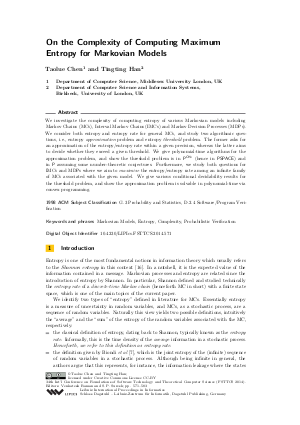Document# On the Complexity of Computing Maximum Entropy for Markovian Models

### Authors Taolue Chen, Tingting Han## File

LIPIcs.FSTTCS.2014.571.pdf
• Filesize: 458 kB
• 13 pages

## Cite As

Taolue Chen and Tingting Han. On the Complexity of Computing Maximum Entropy for Markovian Models. In 34th International Conference on Foundation of Software Technology and Theoretical Computer Science (FSTTCS 2014). Leibniz International Proceedings in Informatics (LIPIcs), Volume 29, pp. 571-583, Schloss Dagstuhl - Leibniz-Zentrum für Informatik (2014)
https://doi.org/10.4230/LIPIcs.FSTTCS.2014.571

## Abstract

We investigate the complexity of computing entropy of various Markovian models including Markov Chains (MCs), Interval Markov Chains (IMCs) and Markov Decision Processes (MDPs). We consider both entropy and entropy rate for general MCs, and study two algorithmic questions, i.e., entropy approximation problem and entropy threshold problem. The former asks for an approximation of the entropy/entropy rate within a given precision, whereas the latter aims to decide whether they exceed a given threshold. We give polynomial-time algorithms for the approximation problem, and show the threshold problem is in P^CH_3 (hence in PSPACE) and in P assuming some number-theoretic conjectures. Furthermore, we study both questions for IMCs and MDPs where we aim to maximise the entropy/entropy rate among an infinite family of MCs associated with the given model. We give various conditional decidability results for the threshold problem, and show the approximation problem is solvable in polynomial-time via convex programming.
##### Keywords
• Markovian Models
• Entropy
• Complexity
• Probabilistic Verification

## Metrics

• Access Statistics
• Total Accesses (updated on a weekly basis)
0